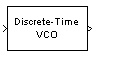Documentation

# Discrete-Time VCO

Implement voltage-controlled oscillator in discrete time

## Library

Components sublibrary of Synchronization## Description

The Discrete-Time VCO (voltage-controlled oscillator) block generates a signal whose frequency shift from the Quiescent frequency parameter is proportional to the input signal. The input signal is interpreted as a voltage. If the input signal is u(t), then the output signal is

`$y\left(t\right)={A}_{c}\mathrm{cos}\left(2\pi {f}_{c}t+2\pi {k}_{c}{\int }_{0}^{t}u\left(\tau \right)d\tau +\phi \right)$`

where Ac is the Output amplitude, fc is the Quiescent frequency, kc is the Input sensitivity, and $\phi$ is the Initial phase

This block uses a discrete-time integrator to interpret the equation above.

This block accepts a scalar-valued input signal with a data type of `single` or `double`. The output signal inherits its data type from the input signal. The block supports double precision only for code generation.

## Parameters

Output amplitude

The amplitude of the output.

Quiescent frequency (Hz)

The frequency of the oscillator output when the input signal is zero.

Input sensitivity

This value scales the input voltage and, consequently, the shift from the Quiescent frequency value. The units of Input sensitivity are Hertz per volt.

Initial phase (rad)

The initial phase of the oscillator in radians.

Sample time

The calculation sample time.